# ANIMAL GENETICS BREEDING Principles of Genetics Population Genetics

• Slides: 17ANIMAL GENETICS & BREEDING Principles of Genetics & Population Genetics Course No. AGB Lecture no. – 5 UNIT - I Regression Dr K G Mandal Department of Animal Genetics & Breeding Bihar Veterinary College, Patna Bihar Animal Sciences University, Patna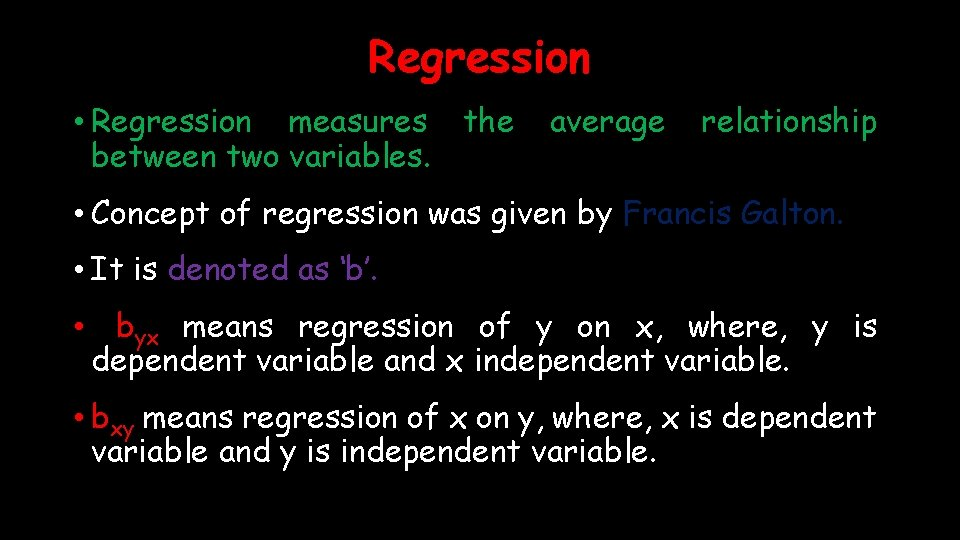Regression • Regression measures between two variables. the average relationship • Concept of regression was given by Francis Galton. • It is denoted as ‘b’. • byx means regression of y on x, where, y is dependent variable and x independent variable. • bxy means regression of x on y, where, x is dependent variable and y is independent variable.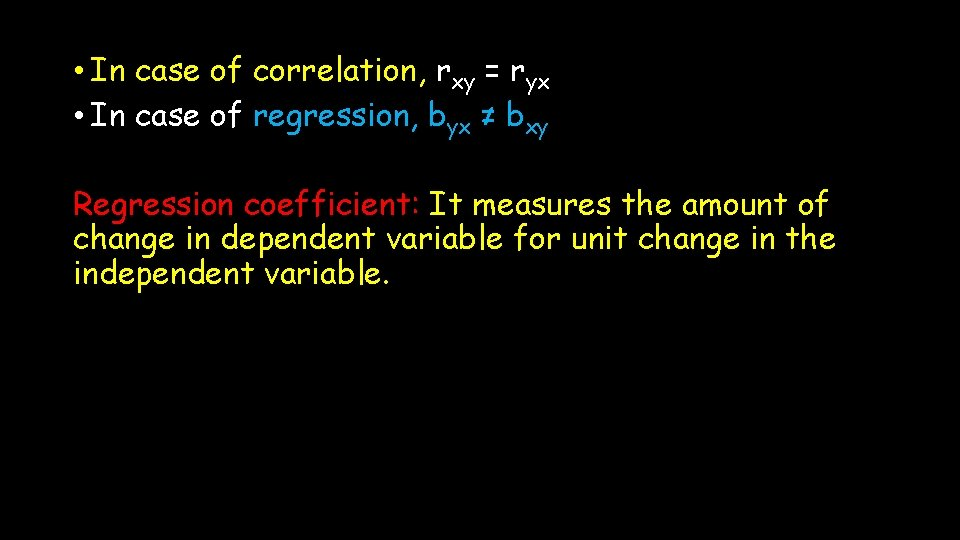• In case of correlation, rxy = ryx • In case of regression, byx ≠ bxy Regression coefficient: It measures the amount of change in dependent variable for unit change in the independent variable.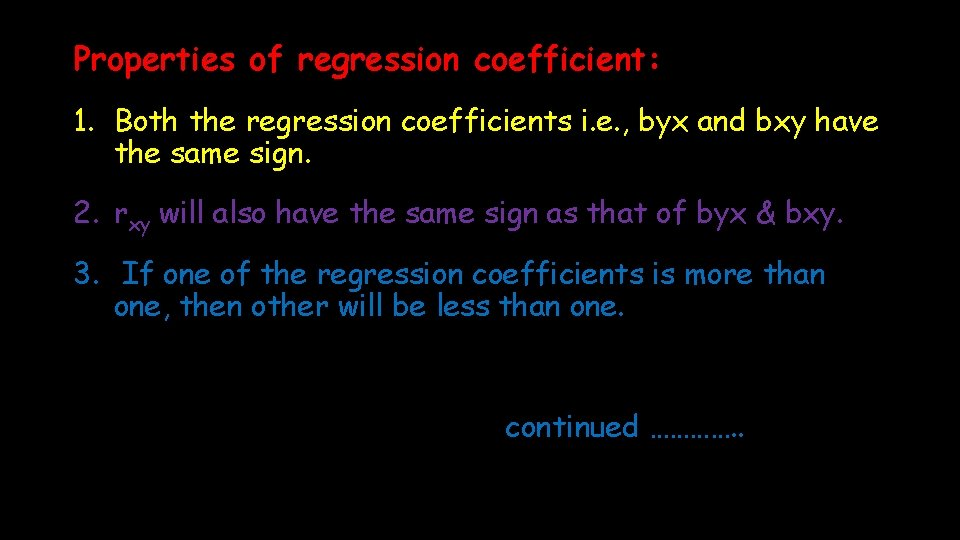Properties of regression coefficient: 1. Both the regression coefficients i. e. , byx and bxy have the same sign. 2. rxy will also have the same sign as that of byx & bxy. 3. If one of the regression coefficients is more than one, then other will be less than one. continued …………. .Regression coefficients have the same unit as that of the unit of original observation. 4. 5. The arithmetic mean of two regression coefficients is more than one [½(bxy + byx) ≥ 1]. 6. The maximum value of bxy. byx = 1 Because bxy. byx = r 2 & maximum value of r = 1, so r 2 = 1.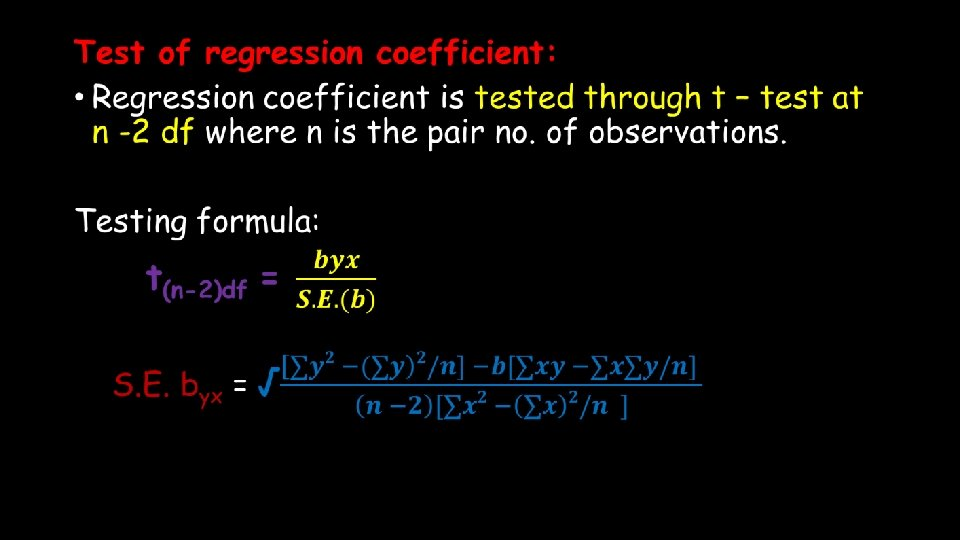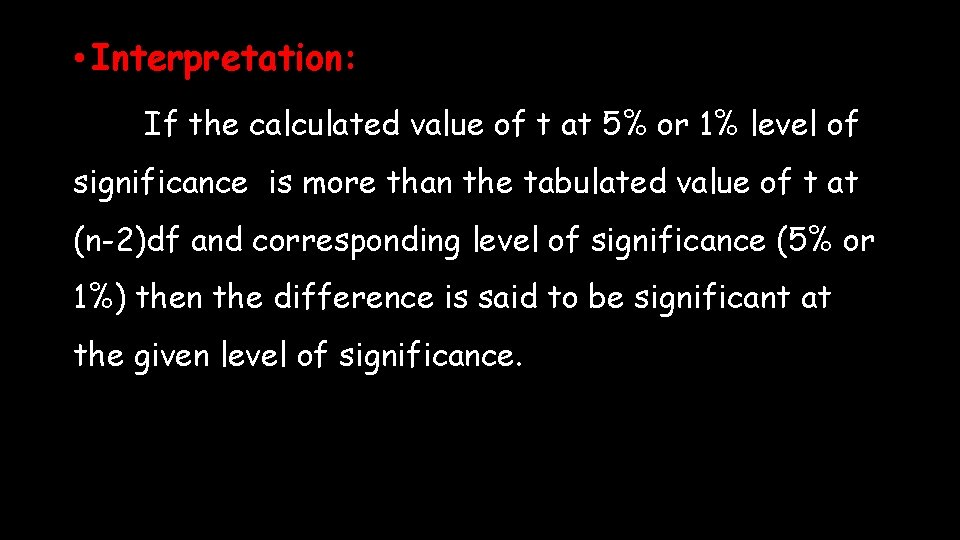• Interpretation: If the calculated value of t at 5% or 1% level of significance is more than the tabulated value of t at (n-2)df and corresponding level of significance (5% or 1%) then the difference is said to be significant at the given level of significance.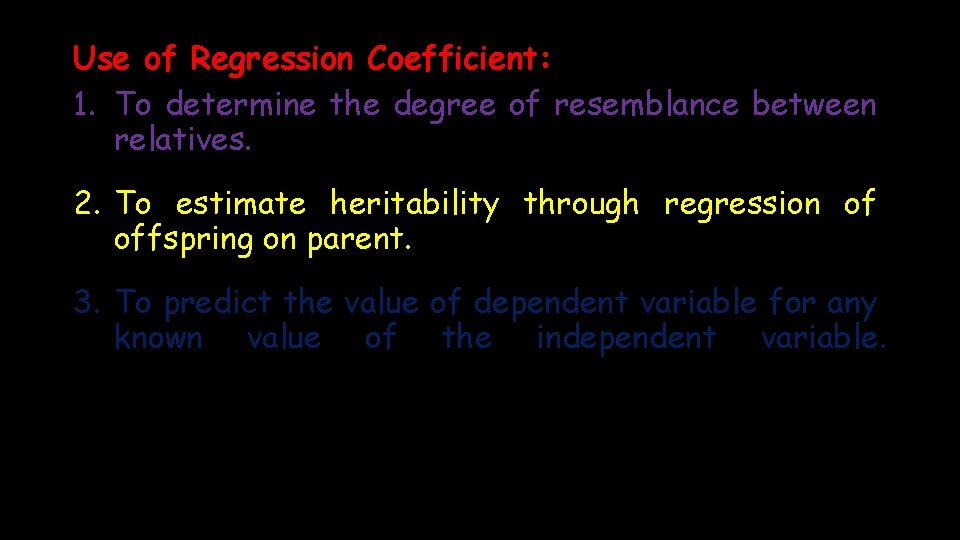Use of Regression Coefficient: 1. To determine the degree of resemblance between relatives. 2. To estimate heritability through regression of offspring on parent. 3. To predict the value of dependent variable for any known value of the independent variable.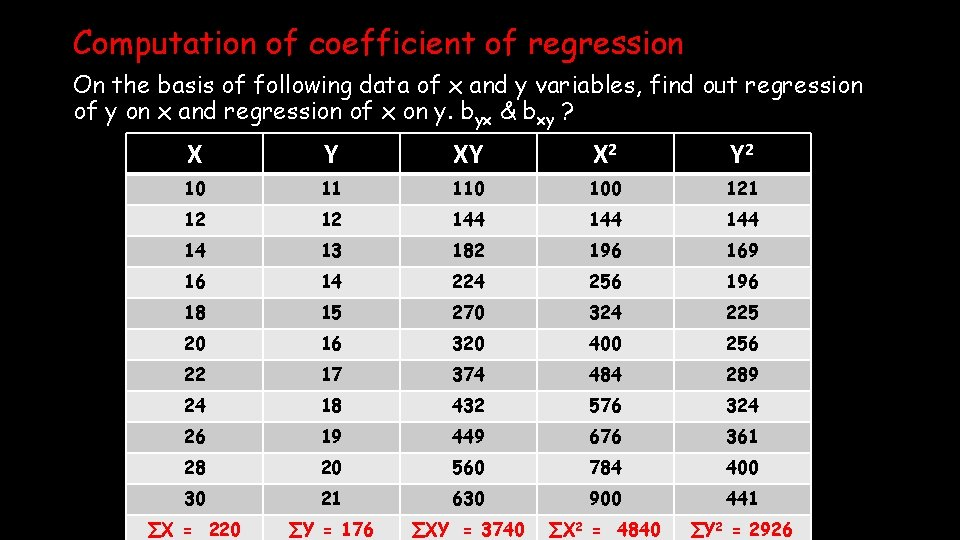Computation of coefficient of regression On the basis of following data of x and y variables, find out regression of y on x and regression of x on y. byx & bxy ? X Y XY X 2 Y 2 10 11 110 100 121 12 12 144 144 14 13 182 196 169 16 14 224 256 196 18 15 270 324 225 20 16 320 400 256 22 17 374 484 289 24 18 432 576 324 26 19 449 676 361 28 20 560 784 400 30 21 630 900 441 ∑X = 220 ∑Y = 176 ∑XY = 3740 ∑X 2 = 4840 ∑Y 2 = 2926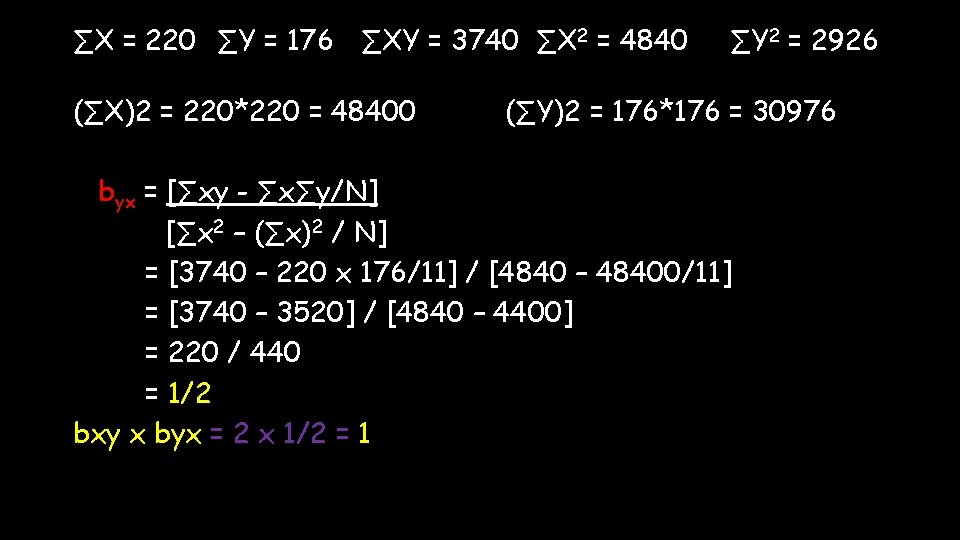∑X = 220 ∑Y = 176 ∑XY = 3740 ∑X 2 = 4840 (∑X)2 = 220*220 = 48400 ∑Y 2 = 2926 (∑Y)2 = 176*176 = 30976 byx = [∑xy - ∑x∑y/N] [∑x 2 – (∑x)2 / N] = [3740 – 220 x 176/11] / [4840 – 48400/11] = [3740 – 3520] / [4840 – 4400] = 220 / 440 = 1/2 bxy x byx = 2 x 1/2 = 1THANK YOU